• 循环结构可以嵌套选择结构吗
2021-08-08 21:18:21

### 循环结构知识整理

###### 上周学习了三个循环结构，分别是while循环、 do while循环 、for循环。基本的循环结构都很容易理解，其中感觉嵌套循环很巧妙，特别是在一些小例题的应用上。

1、第一个小例题是输出一个3行5列的*；
分析：
这种问题很好的解决办法就是用一个嵌套循环，即在一个for循环里套一个for循环，第一个是循环为了规定行的个数，第二个就规定列的个数。
代码如下：

for(var i=0;i<3;i++){
for(var j=0;j<5;j++){
document.write('*&nbsp')
}
document.write("<br/>");
}


注：&nbsp为一个空格；br为换行符号；

2、第二个小例题是用*输出一个直角三角形；
分析：
方法和第一个差不多，将j的取值范围变一下就行

for(i=0;i<=5;i++){
for(j=0;j<i;j++){
document.write('*&nbsp')
}
document.write("<br/>");
}



3、第三个小例题是输出一个99乘法表
分析：
1、首先得找9
9乘法表的规律；
1x1=1
1x2=2 2x2=4
1x3=3 2x3=6 3x3=9
通过观察可以发现从列的角度看第1个数是代表第几列，第2个数则是第几行，通过规律可以把表达式写出来，即：行x列=（行x列）。

代码如下：

for(var i=0;i<=9;i++){
for(var j=1;j<i;j++){
document.write(i+'*'+j+'='+(i*j)+'&nbsp&nbsp');
}
document.write('<br/>');
}

更多相关内容
• 文章目录Java流程控制一、用户交互Scanner二、顺序结构三、选择结构if单选择结构if双选择结构if多选择结构嵌套的if结构switch多选择结构四、循环结构while循环do…while循环for循环五、break & continue Java流程...
• ## 循环结构-嵌套循环

千次阅读 2021-01-03 20:07:06
嵌套循环：将一个循环结构A声明在另一个循环结构B的循环体中,就构成了嵌套循环 外层循环：循环结构B 内层循环：循环结构A 2. 说明 内层循环结构遍历一遍，只相当于外层循环循环体执行了一次 假设外层循环需要执行m...

# 1.嵌套循环

嵌套循环：将一个循环结构A声明在另一个循环结构B的循环体中,就构成了嵌套循环
外层循环：循环结构B
内层循环：循环结构A

# 2. 说明

1. 内层循环结构遍历一遍，只相当于外层循环循环体执行了一次
2. 假设外层循环需要执行m次，内层循环需要执行n次。此时内层循环的循环体一共执行了m * n次
3. 外层循环控制行数，内层循环控制列数
4. 不在循环条件部分限制次数的结构：for(;😉 或 while(true)
5. 结束循环有几种方式？
方式一：循环条件部分返回false
方式二：在循环体中，执行break

# 3.练习

1.遍历100以内的偶数,并计算所有偶数的和及偶数的个数

class DoWhileTest
{
public static void main(String[] args){

//遍历100以内的偶数,并计算所有偶数的和及偶数的个数
int num = 1;
int sum = 0;
int count = 0;
do{
if(num % 2 == 0){
System.out.println(num);
sum += num;
count++;
}
num++;
}while(num <= 100);
System.out.println("偶数的总和是：" + sum);
System.out.println("偶数的总数是：" + count);
}
}


2.从键盘读入个数不确定的整数，并判断读入的正数和负数的个数，输入
为0时结束程序。

import java.util.Scanner;
class ForWhileTest
{
public static void main(String[] args){

Scanner scan = new Scanner(System.in);

int positiveNumber = 0;//记录正数的个数
int negativeNumber = 0;//记录负数的个数

while(true){

int number = scan.nextInt();

//判断number的正负情况
if(number > 0){
positiveNumber++;
}else if(number < 0){
negativeNumber++;
}else{
//一旦执行break,跳出循环
break;
}
}
System.out.println("正数的个数是：" + positiveNumber);
System.out.println("负数的个数是：" + negativeNumber);
}

1.  /*				i(行号)		j(*的个数)
*				1			1
**				2			2
***			3			3
****			4			4
*****			5			5

for(int i = 1;i <= 5;i++){
for(int j = 1;j <= i;j++){
System.out.print("*");
}
System.out.println();
}


4.九九乘法表
1 * 1 = 1
2 * 1 = 2 2 * 2 = 4
。。。
9 * 1 = 9 。。。 9 * 9 = 81

class NineNineTable{
public static void main(String[] args){
for(int i = 1;i <= 9;i++){
for(int j = 1;j <= i;j++){
System.out.print(i + "*" + j + "=" + (i*j) + " ");
}
System.out.println();
}
}
}


5.100以内的所有质数的输出。
质数：素数，只能被1和它本身整除的自然数。–>从2开始，到这个数-1结束为止，都不能被这个数本身整除。
最小的质数是：2

class PrimeNumberTest{
public static void main(String[] args){
boolean isFlag = true;//标识i是否被j除尽，一旦除尽，修改其值
for(int i = 2;i <= 100;i++){//遍历100以内的自然数
for(int j = 2;j < i;j++){
if(i % j == 0){//i被j除尽
isFlag = false;
}
}
if(isFlag == true){
System.out.println(i);
}
//重置isFlag
isFlag = true;
}

}
}


优化输出版本

class PrimeNumberTest1{
public static void main(String[] args){
boolean isFlag = true;//标识i是否被j除尽，一旦除尽，修改其值

long start = System.currentTimeMillis();
for(int i = 2;i <= 100;i++){//遍历100以内的自然数
//优化二：对本身是质数的自然数是有效的
for(int j = 2;j < Math.sqrt(i);j++){

if(i % j == 0){//i被j除尽
isFlag = false;
//break;//优化1，添加break，一旦 有除尽的，表明不是质数，不需要再判断了。只对本身非质数的自然数是有效的
}
}
if(isFlag == true){
System.out.println(i);
}
//重置isFlag
isFlag = true;
}
//获取当前时间距离1970-01-01 00:00:00 的毫秒数
long end = System.currentTimeMillis();
System.out.println("耗费时间为：" + (end - start));
}
}

展开全文java
• 通信技术专业教学资源库 深圳职业技术学院 通信系统SIMULINK仿真平台课程 主讲 杨巧莲 MATLAB程序结构 循环结构 课程团队杨巧莲吴丽春张倩董月秋杨柳 循环结构 1 for 循环变量=初值增量步长终值 循环体 end 开始 ...
• 1.选择结构 if语句 格式1： if (关系表达式) { 语句体; } 其它语句; public static void main(String[] args) { System.out.println("开始"); //定义两个变量 int a = 10; in...

## 1.选择结构

• if语句
格式1： if (关系表达式) {
语句体;
}
其它语句;
public static void main(String[] args) {
System.out.println("开始");
//定义两个变量
int a = 10;
int b = 20;
//需求：判断a和b的值是否相等，如果相等，就在控制台输出：a等于b
if (a == b) {
System.out.println("a等于b");
}
//需求：判断a和c的值是否相等，如果相等，就在控制台输出：a等于c
int c = 10;
if (a == c) {
System.out.println("a等于c");
}
System.out.println("结束");
}


运行结果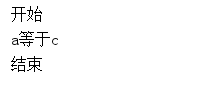• 格式2：
if (关系表达式)
{ 语句体1;
} else {
语句体2;
}
其它语句;
public static void main(String[] args) {
System.out.println("开始");
//定义两个变量
int a = 10; int b = 20; b = 5;
// 需求：判断a是否大于b，如果是，在控制台输出：a的值大于b，否则，在控制台输出：a的值不大于b
if (a > b) {
System.out.println("a的值大于b");
} else {
System.out.println("a的值不大于b");
}
System.out.println("结束");
}


运行结果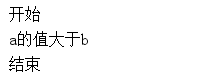-格式3
if (关系表达式1) {
语句体1;
} else if (关系表达式2) {
语句体2;
}

public static void main(String[] args) {
//小明的考试成绩未知，可以使用键盘录入的方式获取值
Scanner sc = new Scanner(System.in);
System.out.println("请输入一个分数：");
int score = sc.nextInt();
//由于奖励种类较多，属于多种判断，采用if...else...if格式实现
// 为每种判断设置对应的条件
// 为每种判断设置对应的奖励
// 数据测试：正确数据，边界数据，错误数据
if (score > 100 || score < 0) {
System.out.println("你输入的分数有误");
} else if (score >= 95 && score <= 100) {
System.out.println("山地自行车一辆");
} else if (score >= 90 && score <= 94) {
System.out.println("游乐场玩一次");
} else if (score >= 80 && score <= 89) {
System.out.println("变形金刚玩具一个");
} else {
System.out.println("胖揍一顿");
}
}

• 选择结构switch语句
switch(表达式) {
case 常量值1:
语句体1;
break;
case 常量值2:
语句体2;
break;

default:
语句体n+1;
break;
}
//其它语句
public static void main(String[] args) {
//定义月份变量，判断季节
int month = 6;
//switch语句实现选择
switch (month) {
case 1:
System.out.println("冬季");
break;
case 2:
System.out.println("冬季");
break;
case 3:
System.out.println("春季");
break;
case 4:
System.out.println("春季");
break;
case 5:
System.out.println("春季");
break;
case 6:
System.out.println("夏季");
break;
case 7:
System.out.println("夏季");
break;
case 8:
System.out.println("夏季");
break;
case 9:
System.out.println("秋季");
break;
case 10:
System.out.println("秋季");
break;
case 11:
System.out.println("秋季");
break;
case 12:
System.out.println("冬季");
break;
default:
System.out.println("你输入的月份数字有误");
break;
}
}


## 2.循环结构

概述
循环语句可以在满足循环条件的情况下，反复执行某一段代码，这段被重复执行的代码被称为循环体语句，当反复执行这个
循环体时，需要在合适的时候把循环判断条件修改为false，从而结束循环，否则循环将一直执行下去，形成死循环。

1. for循环
格式：
for(初始化表达式①; 布尔表达式②; 步进表达式④){
循环体③
}
//其它语句
public static void main(String[] args) {
//需求：输出数据1-5
for (int i = 1; i <= 5; i++) {
System.out.println(i);
}
System.out.println("--------");
//需求：输出数据5-1
for (int i = 5; i >= 1; i--) {
System.out.println(i);
}
}


运行结果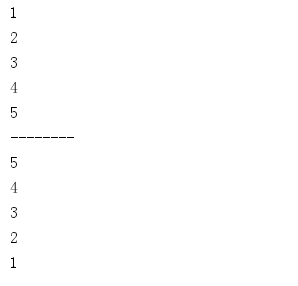2. while循环
格式：
初始化表达式①
while(布尔表达式②){
循环体③
步进表达式④
}
//其它语句

public static void main(String[] args) {
//for循环实现打印10次HelloWorld
for (int i = 1; i <= 10; i++) {
System.out.println("HelloWorld");
}
//while循环实现打印10次HelloWorld //定义初始化变量
int i = 1; //循环条件<=10
while (i <= 10) {
System.out.println("HelloWorld");
//步进
i++;
}
}


运行结果3. do while循环
格式：
初始化表达式①
do{
循环体③
步进表达式④
}while(布尔表达式②);
//其它语句

public static void main(String[] args) {
int x = 1;
do {
System.out.println("HelloWorld");
x++;
} while (x <= 10);
}


运行结果同上

• 循环跳转
break语句：
使用场景：终止switch或者循环
在选择结构switch语句中
在循环语句中
离开使用场景的存在是没有意义的
 public static void main(String[] args) {
for (int i = 1; i <= 10; i++) {
if (i % 5 == 0) {
break;
}
System.out.println("HelloWorld" + i);
}
}


运行结果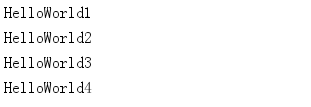continue语句
使用场景：结束本次循环，继续下一次的循环
只能使用在循环语句中

public static void main(String[] args) {
for (int i = 1; i <= 10; i++) {
if (i % 5 == 0) {
continue;
}
System.out.println("HelloWorld" + i);
}
}


运行结果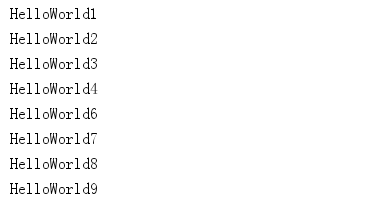## 3.循环嵌套

所谓嵌套循环，是指一个循环的循环体是另一个循环。比如for循环里面还有一个for循环，就是嵌套循环。总共的循环次数=外循环次数*内循环次数

public static void main(String[] args) {
//打印2021年至2023年月份
// 年份是外循环，3年；月份是内循环，12月
for (int i = 2021; i <= 2023; i++) {
for (int j = 1; j <= 12; j++) {
//不换行打印星号
System.out.print(i + "年" + j + "月 ");
}
//内循环打印12个月后，需要一次换行
System.out.println();
}
}


运行结果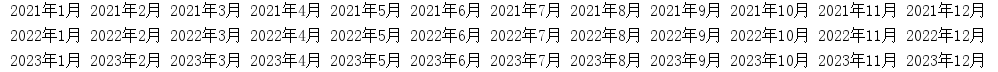展开全文java
• while循环

循环结构又称为重复结构，是利用计算机运算速度快以及能进行逻辑控制得我特点来重复执行默写操作。

while循环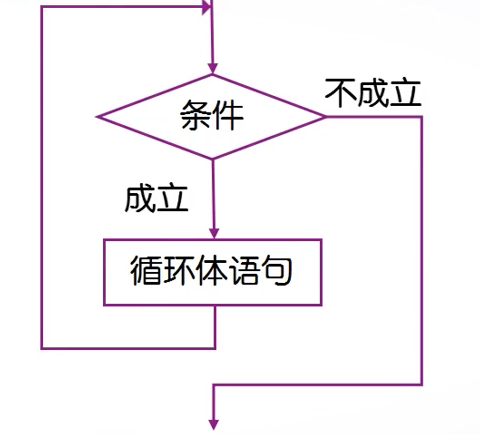while语句多用于循环次数不确定的情况，而对于循环次数确定的情况，使用for语句更方便。

针对不同情况可以选择不同的循环语句，但从功能上将两种循环语句可以相互替代。

以下是一个例子：

musm=0;
n=0;
x=input('Enter a number(end in 0):');
while x~=0
msum=musm+x;
n=n+1;
x=input('Enter a number(end in 0):');
end
if n>0
msum;
mean=msum/n;
end

for语句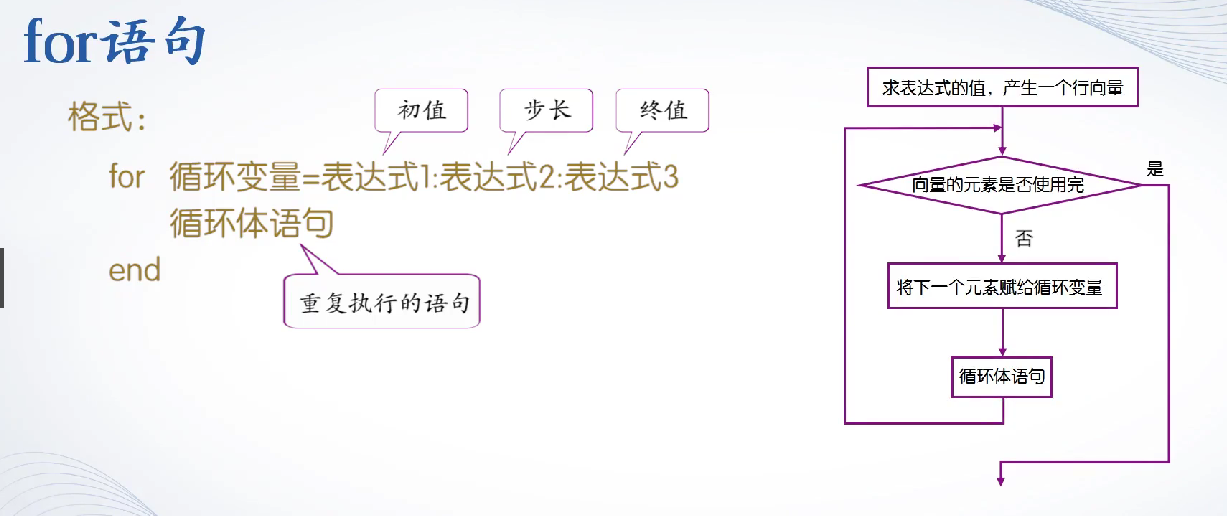• for语句针对向量的每一个元素执行一次循环体

• 退出循环之后，循环变量的值就是向量中最后的元素值

• 当向量不为空时，循环体一次也不执行

用无穷级数算 π

y=0;
g=-1;
n=input('n=');
for i=1:n
g=-g;
y=y+g*1/(2*i-1);
end
format long
pai=4*y;

向量形式表达更加简洁：

n=input('n=');
x=1:2:(2*n-1);
y=(-1).^(2:n+1)./x;
pai=sum(y)*4
format long
n=9999999

pai =

3.141592753589700

定积分求π的近似值

 a=0;
b=1;
n=input('n=');
h=(b-a)/n;
x=a:h:b;
f=sqrt(1-x.*x);
s=[];
for k=1:n
s1=(f(k)+f(k+1))*h/2;
s=[s,s1];
end
pai=4*sum(s)
n=99999

pai =

3.141592616401430


没有办法，内存没那么大，算力不够，迭代这么多次应该也够用

按照MATLAB的定义，for语句的循环结构也可以是一个列向量，其格式为：

for循环变量=矩阵表达式

循环体语句

end

break语句和continue语句

break语句用来跳出循环体，结束整个循环。

continue语句用来结束本次循环，接着进行下一次是否执行循环的判断。

for n=100:200
if rem(n,21)~=0
continue
end
n;
break
end

循环的嵌套

如果一个循环结构的循环体又包含一个循环结构，就称为循环的嵌套，或称为多重循环结构。

这个程序可以找到自然数范围内的素数，我个人觉得最巧妙的是第二个for语句没有把2剔除而可以把2的倍数剔除

m=input('m=');
p=1:m;
p(1)=0;
for i=2:sqrt(m)
for j=2*i:i:m
p(j)=0;
end
end
n=find(p~=0);
p(n)

以上是循环结构的内容

展开全文matlab
• dowhile语句 for语句 循环结构嵌套PPT课件.pptx
• java为我们提供了while do-while for三种循环结构 一.while语法结构： while(条件（布尔类型的）){ // 循环体具体做什么事情 } while循环执行流程： 一个循环可能会循环执行多次 第一次判断条件true，继续执行 第...
• dowhile语句 for语句 循环结构嵌套PPT学习教案.pptx
• 主要介绍了Python 循环嵌套的相关资料，文中示例代码非常详细，帮助大家更好的理解和学习，感兴趣的朋友可以了解下
• 在Java中有三种流程结构：顺序结构，选择结构，循环结构。 if控制语句 if控制语句共有三种不同的形式，分别是单分支结构，双分支结构和多分枝结构。 if语句由条件表达式和紧随其后的语句组成。如果if条件表达式...java
• 第5章 循环结构考点归纳5.1 for循环结构1.for循环语句的一般表达式是：for(表达式1；表达式2；表达式3) 语句2.C语言语法规定：循环体语句只能包含一条语句，若需多条语句，应使用复合语句。5.2 while和do while循环...
• C#循环结构嵌套循环 在一个循环体中包含另一个循环，称为“嵌套循环”。当2个循环嵌套的时候，外层循环体执行一次，内层循环体执行 n 次（ n 是内层循环的次数）。请看下面的例子： 运行结果： 比较代码和...c#
• 介绍Python的循环结构，对于初学者来说能够很好地学习和吸收知识
• 循环结构（while-if嵌套循环结构-while-if嵌套 实例6 # 该实例演示了数字猜谜游戏 number = 7 guess = -1 print("数字猜谜游戏!") while guess != number: guess = int(input("请输入你猜的数字：")) if guess =...
• 三种循环可互相嵌套,层数不限 嵌套循环的执行流程
• 04选择结构循环结构函数定义与使用Python数据分析挖掘与可视化本章学习目标理解条件表达式的值与True或False的等价关系熟练掌握选择结构熟练掌握循环结构理解带else的循环结构执行过程熟练掌握函数的定义与使用熟练...
• 第 5 章 循环结构程序设计 5.1 基本知识点 while 语句的使用格式和注意事项 do-while 语句的使用格式和注意事项 for 语句的使用格式和注意事项 break 和 continue 语句在循环语句中的应用 循环结构嵌套 使用 goto ...
• 精心整理 第 5 章循环结构程序设计 5.1 基本知识点 while 语句的使用格式和注意事项 do-while 语句的使用格式和注意事项 for 语句的使用格式和注意事项 break 和 continue 语句在循环语句中的应用 循环结构嵌套 ...
• 0 引言在python中，我们学习并掌握了for循环嵌套结构，for循环嵌套结构能够帮我们解决身边的一些数学问题1 问题求1！+3！+5！+……+n！的总和。2 方法需要连续两次利用for...算法 python 人工智能 java 机器学习
• js的循环结构语句 循环结构：根据某些给出的条件，重复的执行同一段代码。 循环嵌套：就是在一个循环里面再写一个循环。 固定内容：初始化变量、条件判断、要重复执行的代码、改变初始化变量。 注意：循环要有尽头，...
• 主要内容包括计算机概述、计算机系统的组成、信息化及指标体系、操作系统、程序设计语言、算法简介、数的表示及存储、数据结构简介及顺序结构和选择结构、循环结构、循环的嵌套、算法复杂度分析，枚举算法，递归与...
• ## Python选择结构和循环结构

千次阅读 多人点赞 2020-03-20 23:19:10
选择循环结构中，条件表达式的值只要不是False、0（或0.0、0j等）、空值None、空列表、空元组、空集合、空字典、空字符串、空range对象或其他空迭代对象，Python解释器均认为与True等价。 （1）关系运算符 ...python python入门基础
• 今天小编就为大家分享一篇关于Python之循环结构，小编觉得内容挺不错的，现在分享给大家，具有很好的参考价值，需要的朋友一起跟随小编来看看吧
• 任务制作一个数字电子钟 一任务分析 1目标 制作实际生活中常见的显示时分秒的 数字电子钟如 1011562DigTime.EXE程序演示 3所需知识 顺序结构选择结构循环结构程序设计C语言程序设计教程第二版Lizq98@任务制作一个...
• 麻烦提供书名和作者，感谢】Python3.6之for循环嵌套撰写代码第1个代码 ：foriinrange(0,4):forjinrange(0,7):print("*",end="")print()说明：外循环用变量 i，一共打印4行：即：当i=0时，打印第1行，i=1时，打印第2...
• Java的循环结构嵌套循环Java的循环结构while 循环结构do...while 循环结构for 循环结构Java的嵌套循环概念循环控制语句breakcontinuereturnforeach 循环 Java的循环结构 Java 总共有三种循环结构，分别是while、do...java
• 主要介绍了讲解C++的do while循环循环语句的嵌套使用方法,是C++入门学习中的基础知识,需要的朋友可以参考下...